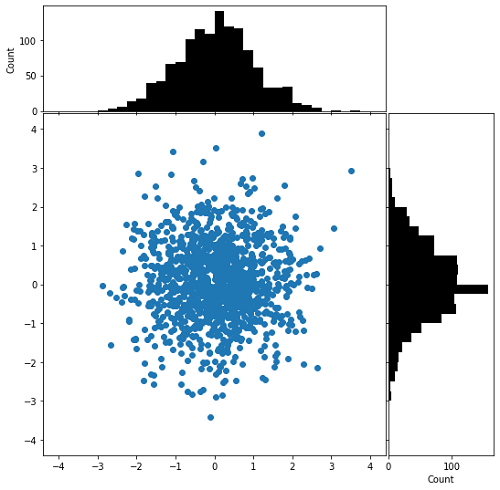# Python | Hist-Scatter Hybrid Plot

In this tutorial, we are going to learn how to hist-scatter plot using matplotlib in Python?
Submitted by Anuj Singh, on August 16, 2020

This is one of the most favored plots by data visualization experts. The scatter points and histograms are joined together and provide a complete picture of each data point. There are three different plots integrated into the complete figure. Following is an example of the implementation of a hist-scatter plot.

Illustrations:## Python code for hist-scatter hybrid plot

```import numpy as np
import matplotlib.pyplot as plt

x = np.random.randn(1230)
y = np.random.randn(1230)

def scatter_hist(x, y, ax, ax_histx, ax_histy):
ax_histx.tick_params(axis="x", labelbottom=False)
ax_histy.tick_params(axis="y", labelleft=False)

ax.scatter(x, y)

binwidth = 0.25
xymax = max(np.max(np.abs(x)), np.max(np.abs(y)))
lim = (int(xymax/binwidth) + 1) * binwidth

bins = np.arange(-lim, lim + binwidth, binwidth)
ax_histx.hist(x, bins=bins, color='black')
ax_histx.set_ylabel('Count')
ax_histy.set_xlabel('Count')
ax_histy.hist(y, bins=bins, orientation='horizontal', color='black')

# definitions for the axes
left, width = 0.1, 0.65
bottom, height = 0.1, 0.65
spacing = 0.005

rect_scatter = [left, bottom, width, height]
rect_histx = [left, bottom + height + spacing, width, 0.2]
rect_histy = [left + width + spacing, bottom, 0.2, height]

fig = plt.figure(figsize=(8, 8))

# use the previously defined function
scatter_hist(x, y, ax, ax_histx, ax_histy)

plt.show()
```

Output:

```Output is as Figure
```

Languages: » C » C++ » C++ STL » Java » Data Structure » C#.Net » Android » Kotlin » SQL
Web Technologies: » PHP » Python » JavaScript » CSS » Ajax » Node.js » Web programming/HTML
Solved programs: » C » C++ » DS » Java » C#
Aptitude que. & ans.: » C » C++ » Java » DBMS
Interview que. & ans.: » C » Embedded C » Java » SEO » HR
CS Subjects: » CS Basics » O.S. » Networks » DBMS » Embedded Systems » Cloud Computing
» Machine learning » CS Organizations » Linux » DOS
More: » Articles » Puzzles » News/Updates